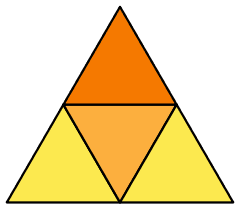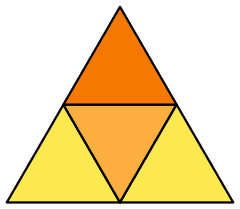# Curvature and locally flat spaces

A curved space and be flattened out locally, giving a flat "subspace" while increasing the curvature around that "subspace", right? If so, wouldn't it be possible to make any curved space flat everywhere and concentrate all the curvature at a single point and move that point infinately far away, creating a space which, for all intents and uses, it flat?

If not, why not?

A curved space and be flattened out locally, giving a flat "subspace" while increasing the curvature around that "subspace", right? If so, wouldn't it be possible to make any curved space flat everywhere and concentrate all the curvature at a single point and move that point infinately far away, creating a space which, for all intents and uses, it flat?

If not, why not?

Indeed, I think you can, why not?
You can transform a semisphere into a cone by concentrating all the curvature into it's vertex, and then the surface will look locally flat, that is, the gaussian curvature of the cone is zero, equal to the plane.
Is that what you asked? If the surface is not compact you can make the vertex go infinitely far, so in the limit, the surface will look all flat.

What about an entire sphere? Is it possible then?

EDIT: More generally, does it work in ANY space?

Last edited:
A curved space and be flattened out locally, giving a flat "subspace" while increasing the curvature around that "subspace", right? If so, wouldn't it be possible to make any curved space flat everywhere and concentrate all the curvature at a single point and move that point infinitely far away, creating a space which, for all intents and uses, it flat?

If not, why not?

One can push curvature onto a set of measure zero - but not a single point in general.
Using the exponential map one gets a diffeomorphism onto an open ball with boundary the conjugate locus. One can splice a flat metric in any smaller ball around the point of exponentiation with the given metric outside of this ball using a partition of unity. Enlarging this ball pushes the region of flatness towards the conjugate locus. i am not sure what happens to the curvature in the limit but it feels like a delta function on the conjugate locus.

Ben Niehoff
Gold Member
So a sphere can be changed into, say, a tetrahedron, where all the curvature is concentrated in the corners.

So a sphere can be changed into, say, a tetrahedron, where all the curvature is concentrated in the corners.

and the edges. I think

and the edges. I think

Well, if we think of curvature as $$\delta\theta=R\delta a$$, where $$\delta\theta$$ is the angular deflection a vector experiences in being parallel transported around a closed loop with area $$\delta a$$ and $$R$$ is the integrated curvature inside the loop, the edges should not have any net curvature as parallel transporting a vector over and edge and back over the same edge again should return it to its initial direction, right? Meanwhile the vertexes will have infinate curvature, as any path around them will give the same deflection of the vector direction.

Ben Niehoff
Gold Member
The edges will have zero curvature. The vertices will have infinite curvature, but finite angular deflection (i.e., the integral of curvature over a region containing the corner). Therefore, the curvature at the vertices will be delta functions.

The edges will have zero curvature. The vertices will have infinite curvature, but finite angular deflection (i.e., the integral of curvature over a region containing the corner). Therefore, the curvature at the vertices will be delta functions.

can you show me a proof?

This is not a proof, but more of a thought experiment.

Here is a map of the surface of the tetrahedron:Image courtesy of Wikipedia. http://en.wikipedia.org/wiki/Tetrahedron

Let's use the curvature definition $$R=\frac{\delta \theta}{\delta a}$$ described above.

We parallel transport a vector around a closed curve on this map, which is plane. Parallel transporting means moving it around a curve keeping it parallel to itself.

we can start in the darkest region and cross over the border into the middle region. Now the vector has gained an angular displacement of $$\arctan(2\sqrt{2})\approx 71^\circ$$.

For the curve to be closed, we need to cross the same border on the way back to the starting point in the darkest region. Doing this cancels the angular displacement the vector gained in going the other way, so it ends up with no angular displacement.

From our definition, $$R=\frac{\delta \theta}{\delta a}=\frac{0}{\delta a}=0$$. So the edges of the tetrahedron has no curvature.

This is not a proof, but more of a thought experiment.

Here is a map of the surface of the tetrahedron:Image courtesy of Wikipedia. http://en.wikipedia.org/wiki/Tetrahedron

Let's use the curvature definition $$R=\frac{\delta \theta}{\delta a}$$ described above.

We parallel transport a vector around a closed curve on this map, which is plane. Parallel transporting means moving it around a curve keeping it parallel to itself.

we can start in the darkest region and cross over the border into the middle region. Now the vector has gained an angular displacement of $$\arctan(2\sqrt{2})\approx 71^\circ$$.

For the curve to be closed, we need to cross the same border on the way back to the starting point in the darkest region. Doing this cancels the angular displacement the vector gained in going the other way, so it ends up with no angular displacement.

From our definition, $$R=\frac{\delta \theta}{\delta a}=\frac{0}{\delta a}=0$$. So the edges of the tetrahedron has no curvature.

right I guessed that is what you meant. what about parallel translating around the corner of a cube? I get pi/4 which seems to be wrong - it should be pi/2 shouldn't it?

Ben Niehoff